# Bit-parallel algorithms for generalized string matching

In the previous post, we looked at how the Shift-AND algorithm can efficiently solve the problem of exact pattern matching. Today, we examine how the same algorithm can be modified for some types of generalized string patterns (i.e. regular expression). In particular, we are interested in patterns with bounded-length runs for arbitrary characters and patterns with optional characters.

# Bounded-length runs for Arbitrary characters

Instead of being literal, the Pattern now contains some flexible-length sequence of arbitrary characters. For instance, take P = bba#(1,3)a. While a and b are regular characters, #(1,3) means any sequence of arbitrary characters with length in the range from 1 to 3. With that, P can match bbaaa, bbabaa, bbacada, etc. In general, #(L,U) represents a run of arbitrary characters with length from L (lower-bound) to U (upper-bound), inclusively.

To facilitate this flexibility, we introduce a special transition, called the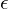-transition. The-transition is special in the sense that it consumes no character. Whenever a state at the input side of the-transition is active, the corresponding state at the output side is also triggered to be active immediately. See the below figure for illustration.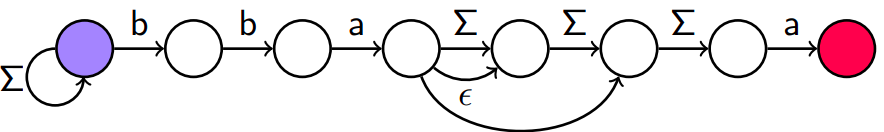The state-transition graph for pattern P = bba#(1,3)a. Notice there are 2 -transitions in the graph. Figure taken from Rahmann’s slides.

It is obvious that if we can model this state-transition graph, we can do the matching for patterns with bounded-length runs for arbitrary characters. Before diving into the computations, let us establish some restrictions:

• No two runs are next to each other. (Since if we have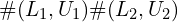, we can merge them into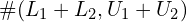.)
• No runs appear at the first or last of the pattern. (Say, for example, if a run is at the first of the pattern, we can just ignore them, then when we find a matching of the truncated pattern, a simple count for the number of characters in the text preceding that matching will solve the problem.)
•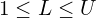. We restrict L to be at least 1 for technical reasons. (If there are 2 runs with a lower bound of 0 are separated by only 1 character, e.g. a#(0,2)b#(0,3)c, the bit-propagation computation may give undesirable results. More details below.)

#### Propagation by subtraction:

Bit-propagation means the process of filling 1 to some determined bits when some conditions are satisfied. For example, in the example (figure) above, since we have 2-transitions, we want the 5-th and the 6-th states (from left to right) are set to 1 when the 4-th state is set to 1.

An important observation: for 2 bitsets X and Y such that:

• X > Y.
• No positions are 1 for both bitsets (i.e. we get 0 if applying AND operation on these 2 bitsets).
• No pair of 1-bits in Y have the same next more significant 1-bit in X. (For example, X = 1000 and Y = 0101 violates this condition since two 1-bits in Y, position 2 and 4, have the same next more significant 1-bit in X, position 1.)

The subtraction X – Y has 1-bit propagated from the subtracted 1-bits to the subtracting 1-bits. Let’s take an example.

We see that X and Y satisfy all the conditions. Then, in the result of their subtraction, X – Y, the positions of 1-bits are either:

• in between a 1-bit in Y (inclusive) and its next more significant 1-bit in X (exclusive). These positions are 2, 3, 8, 9, 10, 12.
• or the 1-bits in X that are chosen by none of the 1-bit in Y as their next more significant 1-bits. In this example, it is in position 5.

With motivation from this observation, we make 2 bitsets of length m, I, and F. While I illustrates the input positions of the-transitions, F marks the positions after the final output of the-transitions. Let us get back to the original example.

The subtraction of F by I (i.e. F – I) gives the bit propagation for the-transitions.

#### The modified Shift-AND algorithm for Bounded-length runs for Arbitrary characters

Given some notations:

• <<: bitset shift to the left.
• |: operator OR.
• &: operator AND.
• ~: inverse operator (0 -> 1, 1 -> 0).
• c: the next character in the text T.
• Step 1: Apply the standard Shift-AND update:

A = ((A << 1) | 1) & M[c]
• Step 2: Propagate the-transitions:

A & I: the active inputs of-transitions.

F – (A & I): all inputs and outputs of active-transitions are set to 1. However, the outputs of inactive-transitions are also 1.

(F – (A & I)) & (~F): deactivate the outputs of inactive-transitions. So, now only the inputs and outputs of active-transitions are set to 1.

A = A | (F – (A & I)) & (~F): update A, completing the bit propagation of active-transitions.

## Patterns with Optional Characters

An example pattern of this type: P = ban?a?na?s. The c? means the pattern can be matched either with or without character c. The-transitions are also useful here, we can plot the transition-state graph as below.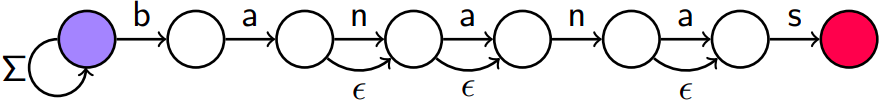The state-transition graph for pattern P = ban?a?na?s. Each optional character is treated as a regular character plus an -transition. Figure taken from Rahmann’s slides.

To match such patterns, we need 3 bitsets:

• I: the bitset of inputs for blocks of consecutive-transitions. Note that for more than 1 optional character standing next to each other, only the first position is set to 1 in I.
• F: the bitset of the final position for each block of consecutive-transitions. For more than 1 optional character standing next to each other, only the last position is set to 1 in F. Note that this is different from the F-bitset for Pattern with Bounded-length runs for Arbitrary characters where F indicates the next character after the last.
• O: the bitset of the output positions of-transitions. Note that for O, we consider each-transition independently rather than in blocks.

The modified Shift-AND algorithm for matching this type of pattern also makes use of some intelligent bitwise-manipulations (the bit-propagation explained in the previous section). The aim of bit-propagation for this problem is: given any block of consecutive-transitions, if any state within a block is active then all states from that point to the end of the block must also be activated.

#### The modified Shift-AND algorithm for Patterns with Optional Characters

For the sake of illustration, let’s consider this simple example:

• A = .0010100.
• I = .0000001.
• O = .1111110.
• F = .1000000.
• Step 1: Apply the standard Shift-AND update:

A = ((A << 1) | 1) & M[c]
• Step 2: Propagate the-transitions:

A | F -> .1010100. : marks the first and the last positions of all propagation blocks. Some in-between states may also be set to 1, but they will be handled later.

(A | F) – I -> .1010011. : For blocks with active states, the not-affected states are flipped from 0 to 1 (i.e. the states that precede the first active state in the block). For blocks with no active states, all states are flipped.

((A | F) – I) = (A | F) -> .1111000. : All states that were flipped in the previous steps are now set to 0, other states are set to 1. So at this moment, all desirable propagating states are set to 1. However, the active states that do not belong to any blocks are also set to 1.

O & (((A | F) – I) = (A | F)) -> .1111000. : keep only the desirable propagating states, the active states that do not belong to any blocks are set to 0.

A | O & (((A | F) – I) = (A | F)) -> .1111100. : bit-propagation is complete.

## Endnotes

The original Shift-AND algorithm is quite simple, yet it can be modified to solve lots of extended problems about pattern matching. Above, we presented two modifications of Shift-AND for patterns with Bounded-length Runs for Arbitrary Characters and patterns with Optional Characters. With some intelligent uses of bit manipulation, we can achieve a linear time complexity for these two problems. Note, however, that we always assume the pattern is short enough to be covered by a word of the Operating System (common word-length at the moment is 64 bits). For longer patterns, we need to distribute the states into multiple words, which might cause an increase in running time.

References:

• Bit-parallel, Sven Rahmann, slides